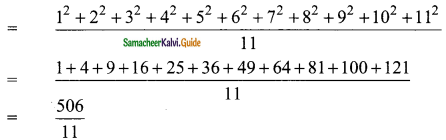Students can download Maths Chapter 8 Statistics Ex 8.4 Questions and Answers, Notes, Samacheer Kalvi 9th Maths Guide Pdf helps you to revise the complete Tamilnadu State Board New Syllabus, helps students complete homework assignments and to score high marks in board exams.

## Tamilnadu Samacheer Kalvi 9th Maths Solutions Chapter 8 Statistics Ex 8.4

Question 1.
Let m be the mid point and b be the upper limit of a class in a continuous frequency distribution. The lower limit of the class is …….
(a) 2m – b
(b) 2m + b
(c) m – b
(d) m – 2b
Solution:
(a) 2m – b

Question 2.
The mean of a set of seven numbers is 81. If one of the numbers is discarded, the mean of the remaining numbers is 78. The value of discarded number is ……..
(a) 101
(b) 100
(c) 99
(d) 98
Solution:
(c) 99
Hint:
Total of 8 numbers = 81 × 7 = 567
Total of 7 numbers = 78 × 6 = 468
The number is = 567 – 468
= 99Question 3.
A particular observation which occurs maximum number of times in a given data is called its ………
(a) frequency
(b) range
(c) mode
(d) median
Solution:
(c) mode

Question 4.
For which set of numbers do the mean, median and mode all have the same values?
(a) 2, 2, 2, 4
(b) 1, 3, 3, 3, 5
(c) 1, 1, 2, 5, 6
(d) 1, 1, 2, 1, 5
Solution:
(b) 1, 3, 3, 3, 5Question 5.
The algebraic sum of the deviations of a set of n values from their mean is ……..
(a) 0
(b) n – 1
(c) n
(d) n + 1
Solution:
(a) 0Question 6.
The mean of a, b, c, d and e is 28. If the mean of a, c and e is 24, then mean of b and d is ……..
(a) 24
(b) 36
(c) 26
(d) 34
Solution:
(d) 34
Hint:
Mean = 28
a + b + c + d + e = 28 × 5 = 140
= 140 …… (1)
But $$\frac{a+c+e}{3}$$ = 24
a + c + e = 72
a + b + c + d + e = 140
b + d + 72 = 140
b + d = 140 – 72
= 68
Mean = $$\frac{68}{2}$$
= 34Question 7.
If the mean of five observations x, x + 2, x + 4, x + 6, x + 8, is 11, then the mean of first three observations is …….
(a) 9
(b) 11
(c) 13
(d) 15
Solution:
(a) 9
Hint:
Mean = $$\frac{x+x+2+x+4+x+6+x+8}{5}$$
11 = $$\frac{5x+20}{5}$$
5x + 20 = 55
5x = 55 – 20
= 35
x = $$\frac{35}{5}$$
= 7
Mean of first 3 observation is = $$\frac{7+9+11}{3}$$
= 9Question 8.
The mean of 5, 9, x, 17 and 21 is 13, then find the value of x.
(a) 9
(b) 13
(c) 17
(d) 21
Solution:
(b) 13
Hint:
Mean = $$\frac{5+9+x+17+21}{5}$$
13 = $$\frac{52+x}{5}$$
65 = 52 + x
x = 65 – 52
= 13

Question 9.
The mean of the square of first 11 natural numbers is
(a) 26
(b) 46
(c) 48
(d) 52
Solution:
(b) 46
Hint:= 46Question 10.
The mean of a set of numbers is $$\bar { X }$$. If each number is multiplied by z, the mean is ……..
(a) $$\bar { X }$$ + z
(b) $$\bar { X }$$ – z
(c) z$$\bar { X }$$
(d) $$\bar { X }$$
Solution:
(c) z$$\bar { X }$$
Hint:
If each observation is multiplied by k, k ≠ 0 then the arithmetic mean is also multiplied by the same quantity.